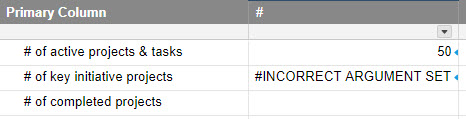# COUNTIFS returning #INCORRECT ARGUMENT SET

Hi all - I cannot figure out what's going wrong here.

I'm trying to count rows in another sheet ("Sheet A") where both the following are true:

• Done = 0
• IsChild = 0

My formula is:

• =COUNTIFS({Sheet A Range 2}, 0, {Sheet A Range 4}, 0)

Now this works perfectly!

So in a cell right below that one, I then try to count rows in Sheet A where both of these criteria are true:

• Done = 0
• Sub-Category = "Key Initiative"

My formula is:

• =COUNTIFS({Sheet A Range 2}, 0, {Sheet A Range 5}, "Key Initiative")

And this doesn't work! It's basically the formula as the above so I'm baffled as to why this one returns an #INCORRECT ARGUMENT SET result while the first one works perfectly? Any ideas?